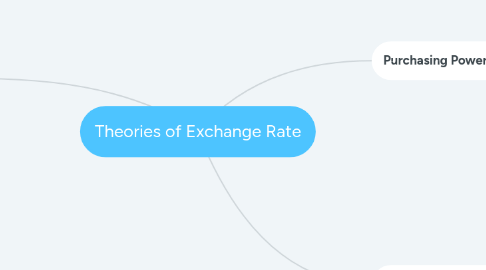# Theories of Exchange RateВасилиса Митрофанова
Get Started. It's FreeTheories of Exchange Rate## 1. Purchasing Power Parity (PPP)

### 1.1. The PPP theory focuses on the inflation- exchange rate relationships. If the law of one price were true for all goods and services, we could obtain the theory of PPP.

1.1.1. Absolute form of PPP

1.1.1.1. The absolute PPP theory postulates that the equilibrium exchange rate between currencies of two countries Is equal to the ratio of the price levels in the two nations. Thus, prices of similar products of two different countries should be equal when measured in a common currency as per the absolute version of PPP theory. (The Law of One price)

1.1.2. Relative form of PPP

1.1.2.1. The relative form of PPP theory is an alternative version which postulates that the change in the exchange rate over a period of time should be proportional to the relative change In the price levels in the two nations over the same time period.